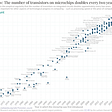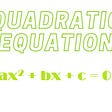# Sum of Squares DOES Factor

Well, not really. The title is just bait to get every Algebra teacher ever to click. Since you are here, give me a few minutes to explain with three common examples.

TL:DR — The truth can be affected by the space of numbers allowed for the coefficients of a polynomial: real or complex. A quadratic sum of squares does not factor if we are limited to real coefficients.

Let’s begin with everybody’s fave — difference of squares.

The idea behind using this special formula is to identify a and b that form the given perfect squares and then substitute.

For example, to factor 49x² − 64y², first identify the a and the b in the formula and then plug in.

We can check by multiplying using the distributive property.

This is your classic factoring problem found in any standardized algebra test ever!

“What is a perfect square? “

Well, Wikipedia says a perfect square is an INTEGER that is the square of another INTEGER. The integers are {…−2, −1, 0, 1, 2…}. Integers are a subset of rational numbers which are fractions of the form a/b where a and b are integers. Of course, b cannot be zero.

Typically in a Beginning Algebra course, we are factoring polynomials under the requirement that the coefficients are rational numbers.

However, most of the polynomials are expressed with integer coefficients for valid reasons I will cover another day.

“What if we do not have a perfect square?”

We can extend the difference of squares formula to factor other “less perfect” binomials. If we allow our polynomials to have any real number coefficients, not just rational numbers, we CAN factor it.

Consider something like 4x² − 5.

Here 5 is not technically a perfect square, but it can be considered the square of a square root.

Oh yes, you can consider any positive real number as a square root of itself squared. In general, for any POSITIVE number b we have

This gives us a formula to factor a binomial of this form.

Yes this IS cool! You can use the difference of squares formula if one of the terms is NOT a perfect square — and it works! For example,

At this point we are allowing our polynomials to have any real number coefficients, not just rational numbers. Note that square root of 5 is irrational.

“What if both a and b are not perfect squares?”

BONUS: This can be extended even further. Let a and b both be positive real numbers:

Ooh… difference of any positive real numbers formula? An exercise for you is to multiply this out to see if it works under the condition that a and b are positive real numbers.

“What if we have a negative number?”

If we allow our polynomials to have any complex number coefficients, not just real numbers, we CAN factor sum of squares.

Consider something like 25x² +9 = 4x² −(−9).

Lets keep it relatively simple, b² = −(bi)² where b is a positive real number. Here is a post covering the definition of complex numbers.

Substituting, we have

Wow. This means we can factor sum of squares using the difference of squares formula where b is the imaginary part of the complex number bi.

Alright, here is a quadratic sum of squares to be factored, 25x² + 4.

Verify the factorization by multiplying.

Sum of squares factors!

Under the conditions that the resulting binomials have rational coefficients, then yes, your algebra teacher was right — sum of squares DOES NOT FACTOR. However, if we expand to the complex numbers it DOES.

And so no, your Algebra teacher did not lie to you!

This just goes to show that Algebra is so rich with subtleties.! If you learned something or liked this please clap and share.

Further Study: Some factoring special binomial notes and my enormous factoring playlist below.

--

--

--

## More from John Redden

Professor and Author

Love podcasts or audiobooks? Learn on the go with our new app.

## Enhancing data transparency with the Pennsylvania Department of Corrections## Writing a multi-file-upload Python-web app with user authentication## Context Filters in Tableau## Strategic Analytical Applications in Organizations## Moore’s Law revisited — How long can it keep going?## Why data science is important## Making predictions are beautiful## Ever since I was a little boy, I have only ever wanted to be a Doctor.## John Redden

Professor and Author

## Matrix Multiplication:## Calculus 101